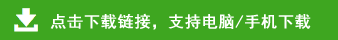# 苏教版小学一年级数学上册20以内加减练习

一年级上学期数学寒假练习

姓名         日期         成绩
6+9=           5+8=        7+3=         6+6=          9+5=
7+9=           8+8=        5+8=         14-9=         17-6=
4+7=           9+4=        6+8=         9+7=          7+8=
9+8=           4+6=        5+3=         6+8=          17-5=
9+1-9=    8+4-8=    5+9-8=     9+9-8=     8+3-8=
6+5-6=    9+1-5=    10+8-7=    7+9-2=    7+8-6=
4+8-4=    8-4+8=    7+9-9=     7-2+9=     3+7-6=
一年级上学期数学寒假练习
姓名         日期         成绩
3+7=           6+8=        9+6=         2+8=          6+9=
14-6=          5+7=        4+7=         7+9=          15-8=
6+9=           8+4=        9+8=         8+5=          9+11=
16-7=          13-7=       7+4=         8+3=          17-6=
7+5-3=    10-6+6=   7+3-4=     2+10-1=    4+7-9=
9+10-7=   7+10-1=   7+5-8=     5+10-7=    9+7-1=
9+2-1=    10-3+5=   7+8-9=     8-2+6=    10-4+4=